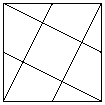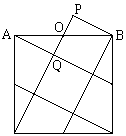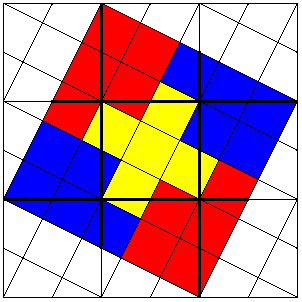# Pleasant math morsels

1. A friend of mine - a mathematician, incidently - recently completed a speed-reading course, and noted this in a letter to his mother. His mother responded with a long, chatty letter in the middle of which she wrote, "Now that you've taken that speed-reading course, you've probably already finished reading this letter."

J. A. Paulos, Mathematics and Humor
The University of Chicago Press, 1980

As you may expect, self-reference appears in a variety of places.2. Lines from the vertices of a square to the midpoints of the sides are drawn, as shown above. Prove the surprising result that the area of the smaller square this produces is 1/5 the given square.

R. Honsberger, Mathematical Morsels
MAA, 1978

The proof appears at the bottom of the page. I have a whole page entirely devoted to simple and beautiful results.## MANIFESTO### ProofAll it takes is to show that the two triangles AQO and BPO are equal. How to complete the proof should be obvious from the diagram.

Another proof, although simple, needs a more complicated diagram. Denote the given square W and assume its area is 1. The small square in question (denoted U) has area say, 1/b. 9 copies of square W form a bigger square.The colored part (say V) is a square that consists of 25 copies of square U and thus its area is 25/b. It is clear from the diagram that the given square is formed by the midlines of square V as square u was formed from square W. We obtain an equation 25/b / b = 1 from which b = 5.

I want to make just one additional remark. Let's return to the original picture of a square with four lines drawn inside it. We may think of identifying opposite sides of the square to obtain a torus. The lines shown will then form a 5 country map, each country being an image of a square. Of course, areas of the countries will by no means be equal.